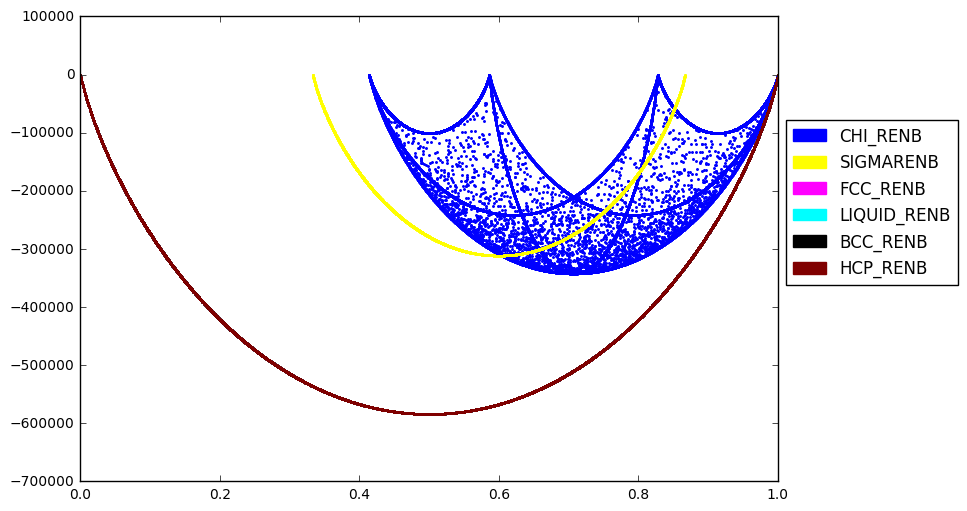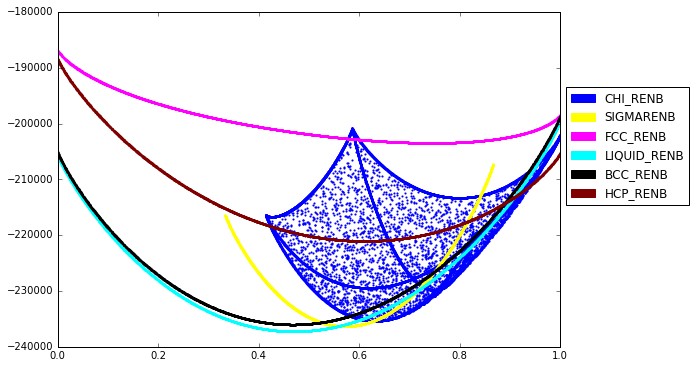{{ message }}

# Calculate gives the wrong result if pressure and temperature are not both specified. #116

Closed
opened this issue Aug 3, 2017 · 3 comments
Closed

# Calculate gives the wrong result if pressure and temperature are not both specified.#116

opened this issue Aug 3, 2017 · 3 comments
Labels
Milestone

###bocklund commented Aug 3, 2017 • edited

 In the binary example, we see the following code produce this plot ```from pycalphad import calculate from pycalphad.plot.utils import phase_legend import numpy as np legend_handles, colorlist = phase_legend(my_phases_nbre) fig = plt.figure(figsize=(9,6)) ax = fig.gca() for name in my_phases_nbre: result = calculate(db_nbre, ['NB', 'RE'], name, T=2800, output='GM') ax.scatter(result.X.sel(component='RE'), result.GM, marker='.', s=5, color=colorlist[name.upper()]) ax.set_xlim((0, 1)) ax.legend(handles=legend_handles, loc='center left', bbox_to_anchor=(1, 0.6)) plt.show()```But changing the temperature to 300 or 1000000 gives the same plot (and the same values from calculate). If you specify pressure in calculate e.g. `result = calculate(db_nbre, ['NB', 'RE'], name, T=2800, P=101325, output='GM')` the resulting plot isFurther changes to temperature produce expected results. The energy surface plotted when P is not specified gives a similar result to T>=6000 (piecewise max in TDB). I assume this is a bug and not the intended result?

###amjokisaari commented Sep 26, 2017

 Please make this throw an error if something isn't specified, instead of silently calculating the wrong thing, as suggested by @richardotis.

###richardotis commented Nov 5, 2017

 In https://github.com/pycalphad/pycalphad/blob/develop/pycalphad/core/calculate.py#L428 The assigned values to `statevar_dict` need to be wrapped with a call to `unpack_condition()` That should fix it.

###richardotis commented Nov 5, 2017

 That does not fix the issue. Still investigating.
added a commit that referenced this issue Nov 5, 2017
``` FIX: Maintain sorted state variable ordering when one or more state v… ```
``` fa08f6a ```
`…ariables is left as default. Fixes gh-116.`
added a commit that referenced this issue Nov 12, 2017
``` FIX: Maintain sorted state variable ordering when one or more state v… ```
``` 0a3e84e ```
`…ariables is left as default. Fixes gh-116.`
added a commit that referenced this issue Nov 12, 2017
``` FIX: Maintain sorted state variable ordering when one or more state v… ```
``` 699468c ```
`…ariables is left as default. Fixes gh-116. (#140)`
added a commit that referenced this issue Nov 26, 2017
``` FIX: Maintain sorted state variable ordering when one or more state v… ```
``` 85c85f5 ```
`…ariables is left as default. Fixes gh-116. (#140)`